NEET  >  31 Year NEET Previous Year Questions: Electromagnetic Induction

# 31 Year NEET Previous Year Questions: Electromagnetic Induction

Test Description

## 25 Questions MCQ Test Physics Class 12 | 31 Year NEET Previous Year Questions: Electromagnetic Induction

31 Year NEET Previous Year Questions: Electromagnetic Induction for NEET 2023 is part of Physics Class 12 preparation. The 31 Year NEET Previous Year Questions: Electromagnetic Induction questions and answers have been prepared according to the NEET exam syllabus.The 31 Year NEET Previous Year Questions: Electromagnetic Induction MCQs are made for NEET 2023 Exam. Find important definitions, questions, notes, meanings, examples, exercises, MCQs and online tests for 31 Year NEET Previous Year Questions: Electromagnetic Induction below.
Solutions of 31 Year NEET Previous Year Questions: Electromagnetic Induction questions in English are available as part of our Physics Class 12 for NEET & 31 Year NEET Previous Year Questions: Electromagnetic Induction solutions in Hindi for Physics Class 12 course. Download more important topics, notes, lectures and mock test series for NEET Exam by signing up for free. Attempt 31 Year NEET Previous Year Questions: Electromagnetic Induction | 25 questions in 50 minutes | Mock test for NEET preparation | Free important questions MCQ to study Physics Class 12 for NEET Exam | Download free PDF with solutions
 1 Crore+ students have signed up on EduRev. Have you?
31 Year NEET Previous Year Questions: Electromagnetic Induction - Question 1

### An inductor may store energy in          

Detailed Solution for 31 Year NEET Previous Year Questions: Electromagnetic Induction - Question 1

An inductor stores energy in its magnetic field.

31 Year NEET Previous Year Questions: Electromagnetic Induction - Question 2

### The current in self inductance L = 40 mH is to beincreased uniformly from 1 amp to 11 amp in 4 milliseconds. The e.m.f. induced in the inductorduring the process is    

Detailed Solution for 31 Year NEET Previous Year Questions: Electromagnetic Induction - Question 2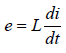Given that L = 40 × 10–3 H,
di = 11 A – 1 A = 10 A
and dt = 4 × 10–3 s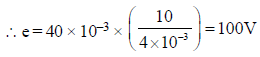31 Year NEET Previous Year Questions: Electromagnetic Induction - Question 3

### A magnetic field of 2 × 10–2 T acts at right anglesto a coil of area 100 cm2, with 50 turns. Theaverage e.m.f. induced in the coil is 0.1 V, when itis removed from the field in t sec. The value of t is

Detailed Solution for 31 Year NEET Previous Year Questions: Electromagnetic Induction - Question 3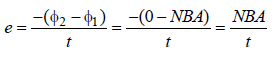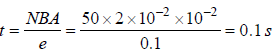31 Year NEET Previous Year Questions: Electromagnetic Induction - Question 4

A 100 millihenry coil carries a current of 1A.Energy stored in its magnetic field is 

Detailed Solution for 31 Year NEET Previous Year Questions: Electromagnetic Induction - Question 4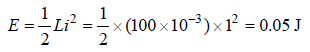31 Year NEET Previous Year Questions: Electromagnetic Induction - Question 5

If the number of turns per unit length of a coil ofsolenoid is doubled, the self-inductance of thesolenoid will 

Detailed Solution for 31 Year NEET Previous Year Questions: Electromagnetic Induction - Question 5

Self inductance of a solenoid =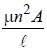So, self induction ∝ n2
So, inductance becomes 4 times when n is doubled.

31 Year NEET Previous Year Questions: Electromagnetic Induction - Question 6

A rectangular coil of 20 turns and area of crosssection 25 sq. cm has a resistance of 100Ω. If amagnetic field which is perpendicular to the planeof coil changes at a rate of 1000 tesla per second,the current in the coil is 

Detailed Solution for 31 Year NEET Previous Year Questions: Electromagnetic Induction - Question 6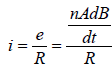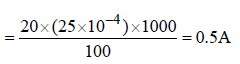31 Year NEET Previous Year Questions: Electromagnetic Induction - Question 7

The total charge induced in a conducting loopwhen it is moved in a magnetic field depend on         

Detailed Solution for 31 Year NEET Previous Year Questions: Electromagnetic Induction - Question 7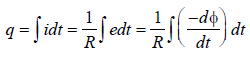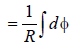(taking only magnitude of e)
Hence, total charge induced in the
conducting loop depends upon the total
change in magnetic flux.

31 Year NEET Previous Year Questions: Electromagnetic Induction - Question 8

What is the self-inductance of a coil whichproduces 5V when the current changes from 3ampere to 2 ampere in one millisecond?       

Detailed Solution for 31 Year NEET Previous Year Questions: Electromagnetic Induction - Question 8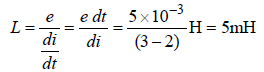31 Year NEET Previous Year Questions: Electromagnetic Induction - Question 9

If N is the number of turns in a coil, the value ofself inductance varies as 

Detailed Solution for 31 Year NEET Previous Year Questions: Electromagnetic Induction - Question 9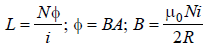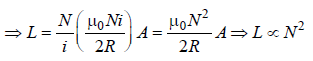31 Year NEET Previous Year Questions: Electromagnetic Induction - Question 10

A conductor of length 0.4 m is moving with aspeed of 7 m/s perpendicular to a magnetic fieldof intensity 0.9 Wb/m2. The induced e.m.f.across the conductor is               

Detailed Solution for 31 Year NEET Previous Year Questions: Electromagnetic Induction - Question 10

Length of conductor (l) = 0.4 m; Speed (v) = 7 m/s and magnetic field (B) = 0.9 Wb/ m2.
Induced e.m.f. (V) = Blv sin θ = 0.9 × 0.4 × 7
× sin 90º = 2.52 V.

31 Year NEET Previous Year Questions: Electromagnetic Induction - Question 11

A varying current in a coil changes from 10A tozero in 0.5 sec. If the average e.m.f induced inthe coil is 220V, the self-inductance of the coil is              

Detailed Solution for 31 Year NEET Previous Year Questions: Electromagnetic Induction - Question 11

Initial current (I1) = 10 A; Final current
(I2)= 0; Time (t) = 0.5 sec and induced e.m.f.
(ε) = 220 V.
Induced e.m.f. (ε)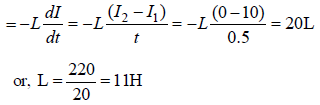[where L = Self inductance of coil]

31 Year NEET Previous Year Questions: Electromagnetic Induction - Question 12

Two coils have a mutual inductance 0.005 H.The current changes in the first coil accordingto equation I = I0 sin ωt, where I0 = 10A and ω = 100π radian/sec. The maximum value of e.m.f.in the second coil is 

Detailed Solution for 31 Year NEET Previous Year Questions: Electromagnetic Induction - Question 12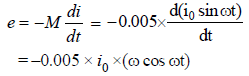emax . = 0.005x i0 xω (when cos ωt = –1)
= 0.005 × 10 × 100π = 5πV

31 Year NEET Previous Year Questions: Electromagnetic Induction - Question 13

In an inductor of self-inductance L = 2 mH,current changes with time according to relationi = t2e–t. At what time emf is zero?              

Detailed Solution for 31 Year NEET Previous Year Questions: Electromagnetic Induction - Question 13

L = 2mH, i = t2e–t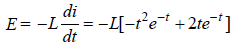when E = 0,
–e–t t2 + 2te–t = 0
or, 2t e–t = e–t t2
⇒t = 2 sec.

31 Year NEET Previous Year Questions: Electromagnetic Induction - Question 14

The magnetic flux through a circuit of resistance R changes by an amount Δφ in a time Δt. Then the total quantity of electric charge Q that passes any point in the circuit during the time Δt is represented by        

Detailed Solution for 31 Year NEET Previous Year Questions: Electromagnetic Induction - Question 14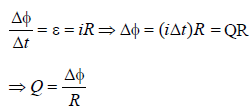31 Year NEET Previous Year Questions: Electromagnetic Induction - Question 15

As a result of change in the magnetic flux linkedto the closed loop shown in the Fig,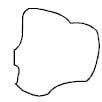an e.m.f. V volt is induced in the loop. The work done (joules) in taking a charge Q coulomb once  along the loop is



Detailed Solution for 31 Year NEET Previous Year Questions: Electromagnetic Induction - Question 15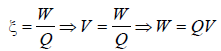31 Year NEET Previous Year Questions: Electromagnetic Induction - Question 16

Two coils of self inductances 2 mH and 8 mH areplaced so close together that the effective fluxin one coil is completely linked with the other.The mutual inductance between these coils is              

Detailed Solution for 31 Year NEET Previous Year Questions: Electromagnetic Induction - Question 16

Mutual Inductance of two coils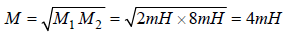31 Year NEET Previous Year Questions: Electromagnetic Induction - Question 17

A long solenoid has 500 turns. When a currentof 2 ampere is passed through it, the resultingmagnetic flux linked with each turn of thesolenoid is 4 ×10–3 Wb. The self- inductance ofthe solenoid is        

Detailed Solution for 31 Year NEET Previous Year Questions: Electromagnetic Induction - Question 17

Total number of turns in the solenoid,
N = 500
Current, I = 2A.
Magnetic flux linked with each turn
= 4 × 10–3 Wb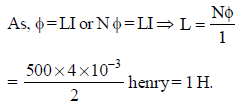31 Year NEET Previous Year Questions: Electromagnetic Induction - Question 18

A rectangular, a square, a circular and an elliptical loop, all in the (x – y) plane, are moving out of a uniform magnetic field with a constant velocity,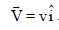The magnetic field is directed along the negative z axis direction. The induced emf, during the passage of these loops, out of the field region, will not remain constant for      

Detailed Solution for 31 Year NEET Previous Year Questions: Electromagnetic Induction - Question 18

The induced emf will remain constant only in the case of rectangular and square loops. In case of the circular and the elliptical loops, the rate of change of area of the loops during their passage out of the field is not constant, hence induced emf will not remain constant for them.

31 Year NEET Previous Year Questions: Electromagnetic Induction - Question 19

A conducting circular loop is placed in a uniformmagnetic field of 0.04 T with its planeperpendicular to the magnetic field. The radiusof the loop starts shrinking at 2 mm/s. The induced emf in the loop when the radius is 2 cmis 

Detailed Solution for 31 Year NEET Previous Year Questions: Electromagnetic Induction - Question 19

Induced emf in the loop is given by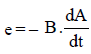where A is the area of the loop.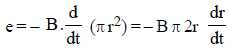r = 2cm = 2 × 10–2 m
dr = 2 mm = 2 × 10–3 m
dt = 1s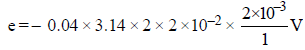= 0.32 π × 10–5V
= 3.2 π ×10–6V
= 3.2 πμV

31 Year NEET Previous Year Questions: Electromagnetic Induction - Question 20

A conducting circular loop is placed in a uniform magnetic field, B = 0.025 T with its plane perpendicular to the loop. The radius of the loop is made to shrink at a constant rate of 1 mm s–1. The induced e.m.f. when the radius is 2 cm, is                             

Detailed Solution for 31 Year NEET Previous Year Questions: Electromagnetic Induction - Question 20

Magnetic flux linked with the loop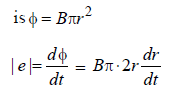When r = 2 cm, dr/dt = 1 mms-1

e = 0.025× π×2 ×2 ×10–2×10–3
= 0.100 × π× 10–5 = π × 10–6 V = πμV

31 Year NEET Previous Year Questions: Electromagnetic Induction - Question 21

The current i in a coil varies with time as shown in the figure. The variation of induced emf with time would be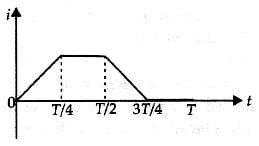Detailed Solution for 31 Year NEET Previous Year Questions: Electromagnetic Induction - Question 21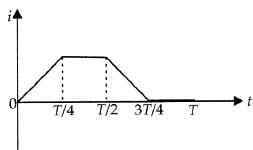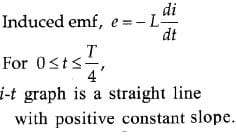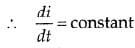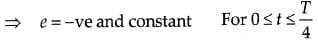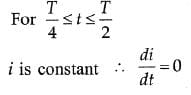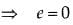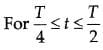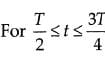i-t graph is a straight line with negative constant slope.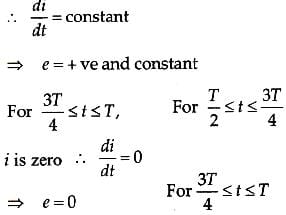From this analysis, the variation of induced emf with time as shown in the figure below.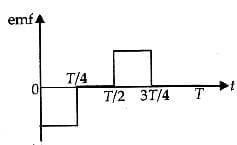31 Year NEET Previous Year Questions: Electromagnetic Induction - Question 22

A coil of resistance 400Ω is placed in a magneticfield. If the magnetic flux φ(wb) linked with thecoil varies with time t (sec) as φ = 50t2 + 4. Thecurrent in the coil at t = 2 sec is : 

Detailed Solution for 31 Year NEET Previous Year Questions: Electromagnetic Induction - Question 22

According, to Faraday’s law of induction
Induced e.m.f.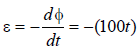Induced current i at t = 2 sec.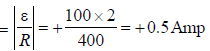31 Year NEET Previous Year Questions: Electromagnetic Induction - Question 23

In a coil of resistance 10 Ω, the induced current developed by changing magnetic flux through it, is shown in figure as a function of time. The magnitude of change in flux through the coil in Weber is :          [2012M]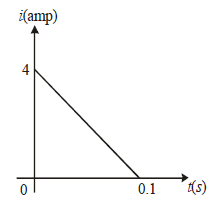Detailed Solution for 31 Year NEET Previous Year Questions: Electromagnetic Induction - Question 23

The charge through the coil = area of
current-time(i – t) graph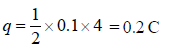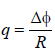∵ Change in flux (Δφ ) = q × R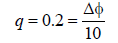Δφ = 2 Weber

31 Year NEET Previous Year Questions: Electromagnetic Induction - Question 24

A wire loop is rotated in a magnetic field. Thefrequency of change of direction of the inducede.m.f. is [NEET 2013]

Detailed Solution for 31 Year NEET Previous Year Questions: Electromagnetic Induction - Question 24

This is the case of periodic EMI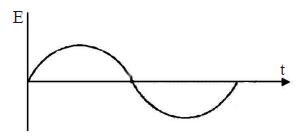From graph, it is clear that direction is
changing once in 1/2 cycle.

31 Year NEET Previous Year Questions: Electromagnetic Induction - Question 25

A current of 2.5 A flows through a coil ofinductance 5 H. The magnetic flux linked withthe coil is [NEET Kar. 2013]

Detailed Solution for 31 Year NEET Previous Year Questions: Electromagnetic Induction - Question 25

Given: current I = 2.5 A
Inductance, L = 5H
Magnatic flux, φ=?
We know, φ = LI ⇒ 5 × 2.5 Wb = 12.5 Wb

## Physics Class 12

157 videos|452 docs|213 tests
Information about 31 Year NEET Previous Year Questions: Electromagnetic Induction Page
In this test you can find the Exam questions for 31 Year NEET Previous Year Questions: Electromagnetic Induction solved & explained in the simplest way possible. Besides giving Questions and answers for 31 Year NEET Previous Year Questions: Electromagnetic Induction, EduRev gives you an ample number of Online tests for practice

## Physics Class 12

157 videos|452 docs|213 tests

### How to Prepare for NEET

Read our guide to prepare for NEET which is created by Toppers & the best Teachers(Scan QR code)